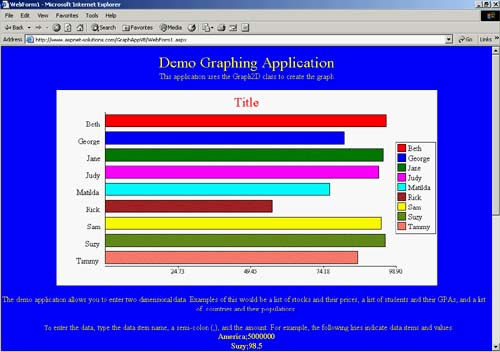# Enhancing the Graph2D Class

### Enhancing the Graph2D Class

I'd like to present two enhancements that you might consider for your version of the Graph2D class. The first enhancement is the ability to guarantee unique file names, even in high-traffic situations. The next is the ability to change the type of graph that's rendered.

To ensure a unique graph number, even for high-volume use, replace the following:

` Dim strName As String = Convert.ToString(DateTime.Now.Ticks) `

with

` Lock() If Application("GraphNumber") = nothing Then   Application("GraphNumber") = 0 End If Dim strName As String = Convert.ToString(Application("GraphNumber")) Application("GraphNumber") = _   Convert.ToInt32(Application("GraphNumber")) + 1   ' Wrap if we get the 1000000.   If Application("GraphNumber") > 1000000 Then     Application("GraphNumber") = 0   End If Unlock() `

I mentioned earlier in this chapter that I use the Graph2D class in an application that I currently support. One of the ways I added some dazzle for the human resources people who use it was to allow them to see a vertical bar graph, a horizontal bar graph, a line graph, and a pie graph. I'm including the horizontal rendering here in Listing 10.14 so that you can see what you need to do to add additional renderings. I would have included all the renderings from my application in this class, but my class requires some specific implementation dependencies.

You can see the horizontal bar graph in Figure 10.6.

##### Listing 10.14 These Methods Make It Possible to Render the Graph with Horizontal Bars Rather Than Vertical Bars.
` ' Draw the bounding lines (left and bottom). Private Sub DrawBounds2(ByVal g As Graphics)     Dim nChartBottom As Integer = m_nHeight - 70     ' Draw the left and bottom lines     g.DrawLine(m_objBlackPen, 100, 45, 100, m_nHeight - 70)     g.DrawLine(m_objBlackPen, 100, m_nHeight - 40, _       m_nLegendX, m_nHeight - 40) End Sub ' Draw the data bars. Private Sub DrawData2(ByVal g As Graphics)     Dim nChartBottom As Integer = m_nHeight - 70     ' Figure out the max value.     m_dMaxValue = 0     Dim nMaxIndex As Integer = 0     Dim i As Integer     For i = 0 To m_nCategoryCount - 1         If m_dMaxValue < m_dDataValues(i) Then             m_dMaxValue = m_dDataValues(i)             nMaxIndex = i         End If     Next     Dim nBarHeight As Integer = ((m_nHeight - 85) / m_nCategoryCount)     For i = 0 To m_nCategoryCount - 1         Dim nBarWidth As Integer = _           (m_dDataValues(i) * (m_nLegendX - 100 - 20)) / m_dMaxValue         Dim x As Integer = 100         Dim y As Integer = 50 + i * nBarHeight         If nBarWidth > 0 Then             g.FillRectangle(New SolidBrush(m_Colors(i And 15)), _                 x, y, nBarWidth, nBarHeight - 10)             g.DrawRectangle(m_objBlackPen, _                 x, y, nBarWidth, nBarHeight - 10)         End If         Dim s2 As SizeF = _             g.MeasureString(m_DataNames(i), m_objLegendFont)         g.DrawString(m_DataNames(i), m_objLegendFont, m_objBlackBrush, _             x - s2.Width - 5, y + (s2.Height / 2))     Next End Sub ' Draw the scale to the left. Private Sub DrawScale2(ByVal g As Graphics)     Dim dDivisor As Double = 1     Dim strModifier As String = ""     ' Billions...     If m_dMaxValue >= 1000000000 Then         strModifier = "B"         If m_dMaxValue >= 100000000000 Then             dDivisor = 100000000000         ElseIf m_dMaxValue >= 10000000000 Then             dDivisor = 10000000000         Else             dDivisor = 1000000000         End If         ' Millions...     ElseIf m_dMaxValue >= 1000000 Then         strModifier = "M"         If m_dMaxValue >= 100000000 Then             dDivisor = 100000000         ElseIf m_dMaxValue >= 10000000 Then             dDivisor = 10000000         Else             dDivisor = 1000000         End If         ' Thousands...     ElseIf m_dMaxValue >= 1000 Then         strModifier = "K"         If m_dMaxValue >= 100000 Then             dDivisor = 100000         ElseIf m_dMaxValue >= 10000 Then             dDivisor = 10000         Else             dDivisor = 1000         End If     End If     Dim nStep As Integer = ((m_nLegendX - 100) / 4)     Dim objScaleFont As New Font("Times New Roman", 9)     Dim i As Integer     For i = 0 To 3         g.DrawLine(m_objBlackPen, 100 + (4 - i) * nStep, _           m_nHeight - 40, 100 + (4 - i) * nStep, m_nHeight - 43)         Dim dThisNumber As Double = _           ((m_dMaxValue / dDivisor) / 4) * (4 - i)         Dim strNumber As String = _           dThisNumber.ToString("0.00") + strModifier         Dim sz As SizeF = g.MeasureString(strNumber, objScaleFont)         g.DrawString(strNumber, objScaleFont, m_objBlackBrush, _           100 + (4 - i) * nStep - sz.Width / 2, m_nHeight - 46)     Next End Sub `
##### Figure 10.6. The Bars Can Go Horizontally with Some Modification to the Code.ASP. NET Solutions - 24 Case Studies. Best Practices for Developers
ISBN: 321159659
EAN: N/A
Year: 2003
Pages: 175